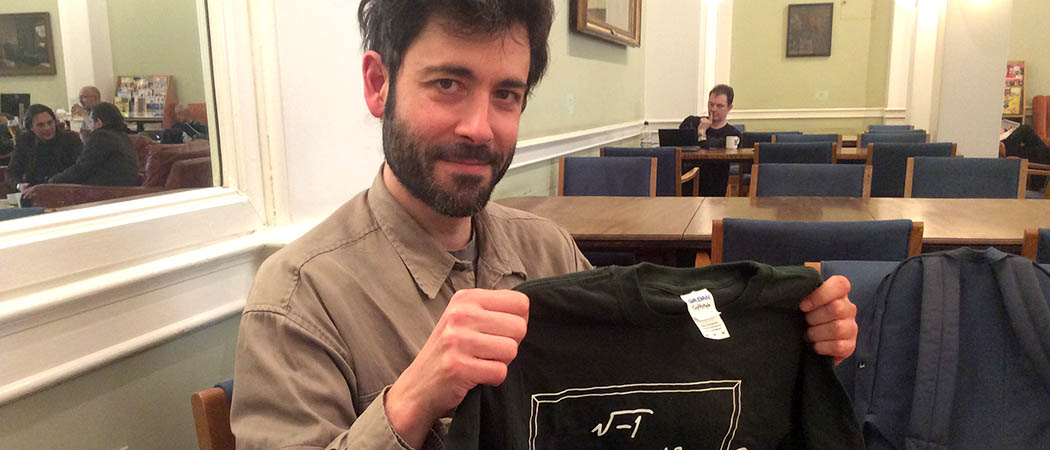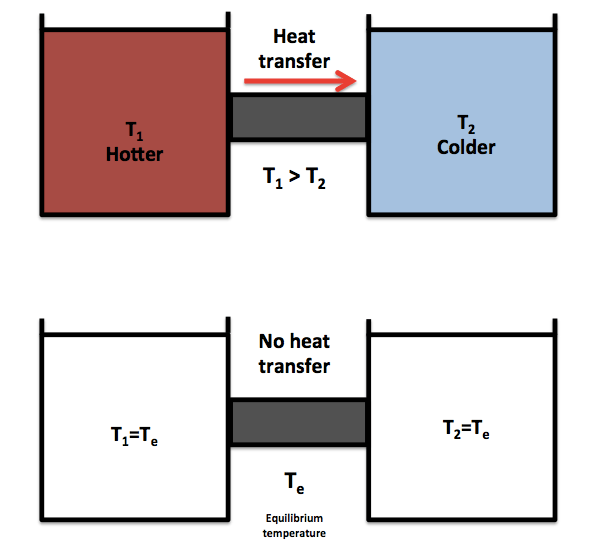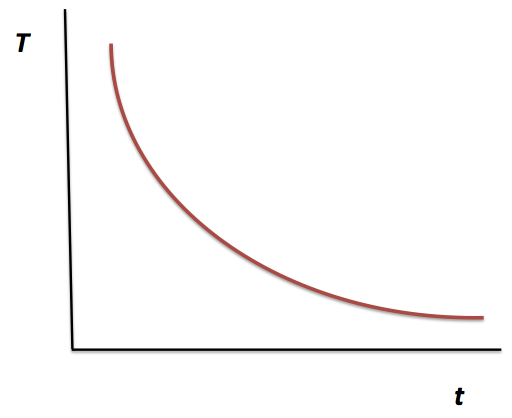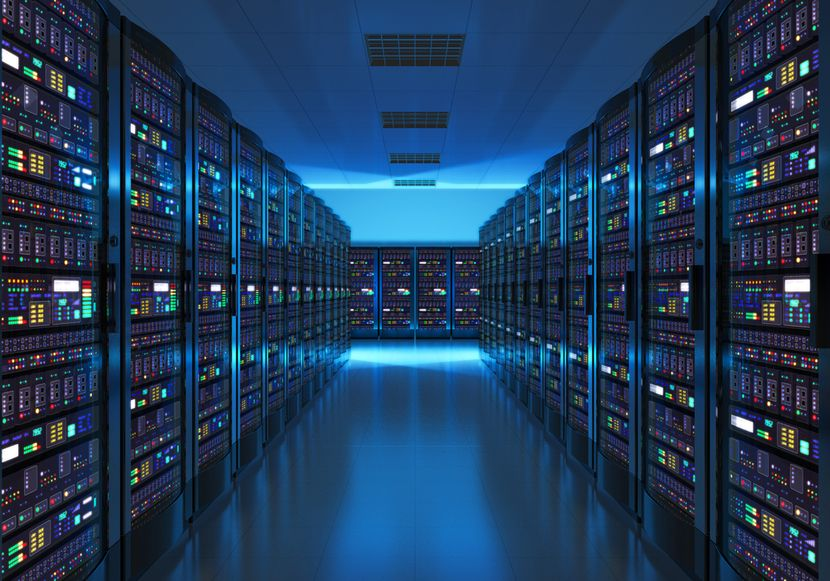# Is it possible to reach absolute zero?

After 100 years, a key postulate of the third law of thermodynamics has been proven. We meet Lluis Masanes, one of the researchers responsible.Lluis Masanes shows off his fashionable Chalkdust T-shirt

The third law of thermodynamics (sometimes referred to as the heat theorem or unattainability principle) was postulated in 1912 by the German chemist Walther H Nernst and states that it is impossible to reach, by any procedure, the coldest temperature possible: absolute zero (0K, or −273.15C or −459.67F, depending on your preferred choice of units). However, this statement was a matter of some controversy, as there was no real proof of it. Although Nernst spent many years defending his version, many scientists refused to accept it, including such heavyweights as Max Planck and Albert Einstein. Both then started introducing their own versions. Einstein, for example, believed that the third law of thermodynamics must rely on the principles of quantum mechanics.

More than one hundred years went by and physicists and chemists from around the world were still debating the theorem, with some remaining unconvinced of its validity, given the lack of a proof.

However, a recently published paper in Nature Communications aims to clarify this debate. Researchers from the Department of Physics and Astronomy at University College London (UCL) have proven mathematically that it is impossible to reach absolute zero: we went to chat with one of them, Lluis Masanes.

Lluis Masanes is one of the co-authors of the recently published paper, A general derivation and quantification of the third law of thermodynamics, and he talked to us about his research, quantum computers and how this work might be applied in the future, especially in the development and design of new cooling methods. But in order to understand the relevance of this achievement, let’s first briefly introduce and explain some of the basic concepts.

## Thermodynamics

Thermodynamics is a branch of physics that describes natural processes involving the transformation of energy, changes in temperature, and the relationship between work and heat. This science is governed by four classical laws (just as Newton’s laws in classical mechanics or Maxwell’s equations in electromagnetism). The four laws of thermodynamics are strongly related to each other, and have been used for hundred of years in the development of engines and cooling processes, in the study of chemical reactions and phase transitions. The results of thermodynamics are also essential for other fields such as physics, chemistry, chemical engineering and aerospace engineering, among others. As in most of the sciences, a background in mathematics is required for its study.

## Brief description of the laws of thermodynamics

#### Zeroth and first laws

The first law of thermodynamics is called the zeroth law of thermodynamics, which is a slightly bizarre number to choose: why didn’t they just call it the first law?  What happened is that when the three laws of thermodynamics were established, they realised that another law was needed to complete the set, but it could not be named the fourth law, as it was the seed of the other three laws and therefore had to be at the head of the list. They also could not renumber the laws, as they were already well known by the scientific community, and this might have lead to confusion.

The zeroth law of thermodynamics, in a few words, defines the concept of temperature. It introduces the idea of thermal equilibrium as well: when two objects are in thermal equilibrium they are said to have the same temperature. The details of the process of reaching this thermal equilibrium are described by the first law (the real first law!) and the second law of thermodynamics.

The first law of thermodynamics (which I think most people are more familiar with) is simply the conservation of energy. It defines a relationship between the forms of energy ($U$) present in a system (the most common being kinetic and potential energy), the work done by the system and heat transfer.Two bodies with different temperatures are placed into contact with each other. In the equilibrium, both have the same temperature and there is no heat transfer between the two.

#### Second law

The second law is included in the book Seventeen equations that changed the world by Ian Stewart. It is used in many fields of science and has led to the development of many important technologies. However, the second law is not as simple to explain as the previous ones. Some examples will paint a general picture. Let’s assume that we have two bodies, one of which is hotter ($T_1$) and the other colder ($T_2$), and therefore $T_1 > T_2$. If we bring both objects into contact, we all would predict that the hotter object would cool down ($T_1$ decreases) and the colder object would heat up ($T_2$ increases), until the thermal equilibrium has been reached.

But we ask ourselves a question: why can it not be the other way around? Why is it not possible to have a scenario where the hot object becomes hotter and the cold one gets even colder? This scenario might not violate either the zeroth or the first law, but for some reason we don’t encounter such a system in real life. How can we explain this? This is where the second law comes into play, and the concept of entropy is introduced.

The entropy measures the number of ways the atoms (and the energy they carry with themselves) can be arranged. According to the second law of thermodynamics, every object has a given amount of entropy associated with it, and whatever happens to it (be it a physical or chemical transformation, phase change, etc) can never result in a decrease in the amount of entropy (for an isolated system). Mathematically speaking, the second law is expressed as:

$S_f \geq S_i$,

where $S_f$ and $S_i$ are the final and initial entropies of the system, respectively. The above equation can also be expressed as a differential, giving the more well-known form of the law:

$\mathrm{d}S \geq 0.$

The entropy remains constant for a reversible process, but is greater than zero for an irreversible process. Taking again the example of putting into contact the hot and cold objects, they will eventually reach the thermal equilibrium (zeroth law). If we then separate both objects, both will remain at the equilibrium temperature $T_e$ (they will not return to their original temperatures $T_1$ and $T_2$). This is an irreversible process, which means that there has been an increase in the total entropy of the system.

Another example of the second law is the following: if you put ice cubes into a glass of water, there will be an increase in the entropy of the total system. When water is in its liquid state, there are many more ways for the water molecules to arrange themselves than when it is in its solid state.

Ludwig Boltzmann explained that entropy could also be interpreted as what’s probable in nature: low-entropy objects are tidy, and unlikely to exist. On the other hand, high-entropy objects are untidy and that makes them more likely to exist. Boltzmann introduced a simple, but important equation to calculate the entropy of any system:

$S=k\ln{W}$,

where $k$ is the Boltzmann constant ($k=1.38064\times10^{-23} \text{m}^2\text{kg s}^{-2} \text{K}^{-1}$) and $W$ is the number of macroscopic configurations that a thermodynamic system can have under determined values of thermodynamic variables (temperature, pressure, volume, etc).

#### Third law

At the beginning of the article, we gave a short introduction to the third law. Here we will use simple maths to understand it better.  Nernst, who we previously read about, conjectured two basic ideas that, taken together, make up the third law of thermodynamics. The first one is formally called the unattainability principle and states that absolute zero is physically unreachable. It was this principle that was under debate and was only proved this past week (we will talk about it shortly, I promise).

The other idea (known as the heat theorem) states that at absolute zero, the entropy of a perfect crystal approaches zero. Under these conditions, one can know the alignment of a perfect crystal exactly, as there is no movement of the particles: there can be just one, unique configuration. Using the equations above, the entropy of the crystal is zero, as $W=1$ (the only configuration possible) and so

$S_0 = k\ln{W}=k\ln{(1)}=0.$

The relevance of this idea is that it provides us with a ground state. If we know the entropy of a system under determined conditions, we can know the entropy in totally different conditions:

$S_1-S_0=\mathrm{d}S \geq 0.$

The change of entropy d$S$ in a system can be calculated using thermodynamic equations (something we will not discuss here); the second law tells us that d$S$ must be greater than zero; we know that energy must be conserved (first law), that there must be a thermal equilibrium (zeroth law); and the third law provides us with information about the entropy at the ground state. We then can calculate $S_1$ for any conditions. With this information, we can solve any thermodynamic problem.

## Interview with Lluis Masanes

Lluis Masanes is a researcher in the Department of Physics and Astronomy at UCL. In collaboration with Jonathan Oppenheim, they mathematically proved the unattainability principle, conjectured by Nerst more than one hundred years ago. They showed that you can’t actually cool a system to absolute zero with a finite amount of resources in a finite time.

We chatted for some minutes with Lluis Masanes about this achievement, and he explained to us the importance of his findings for the design of new cooling processes that might be used in the development of quantum computers. “We found a relation between how low the final temperature is and how much you have to wait” says Masanes. The relation between these two variables, according to Masanes’ research, adopts the form

$T \geq f/t^{7},$

where $T$ is temperature, $t$ is time and $f$ is a function that depends on the characteristics of the cooling machine (for instance a refrigerator, a heat exchanger, etc).Cooling process: The temperature will never reach zero in a finite time according to Masanes’ work

When asked what mathematical techniques he and Oppenheim used, he said that “the mathematical techniques are very simple. It is just about having the right perspective.”

A cooling process can be seen as a series of steps: energy is removed from a system and it is dumped into the environment (a reservoir) again, and again, and again. Each time this is done, the system gets colder and colder, but it becomes harder to cool at each step. How cold it gets depends on how much work can be done to remove the energy and the size of the reservoir for dumping the heat. Masanes says that “if you want to cool a system in a finite amount of time, you cannot interact with an infinite environment”. He continued: “if you have finite time, you cannot put into your system an infinite amount of work”. He then conclude that “finite time implies finite amount of work. Once you have this idea, proving the theorem is easy. You don’t need special or complex techniques.”

#### Analogy of cooling/resetting and quantum computers

Lluis is interested in quantum information theory and its connections to other fields. This was crucial in proving the unattainability principle and defining the limits mentioned above.

The main idea comes from quantum information theory, which he defines as “something that helps us to understand how you can exploit quantum mechanics to process information”.  A cooling process can be thought as resetting or erasing information. A cold system has much lower energy and can be arranged itself into few states. On the other hand, particles can be organised in many more configurations in systems with more energy (hot ones), which makes it very difficult to know what state they settle down to.

“At absolute zero, you know exactly at which state the system will be and that is like erasing information.” He added: “when you erase the information in your computer, the computer has only one state: zero information. Cooling is exactly the same, resetting information”. Erasing information and cooling is about lowering entropy, something that he considers the “most difficult thing”.

The second law has been successfully used in quantum information theory, but the third law was not as simple to use as the second. We asked about the future applications of his research, and he said that “once you know what the limits are, you can’t exceed them” and “this can lead to the design of new cooling methods”.A collection of computer servers, known as a server farm, requires a large amount of power to run and to keep cool. [laboratorio linux CC-BY 2.0]

We also asked him his opinion about quantum computers and how his research can help to develop them. “Our cellphones are classical computers. If we could build computers exploiting the laws of quantum mechanics, they will be much more powerful.” One of the main differences between a classical and a quantum computer is that the basic unit of information in the former is the bit, which has two possible states, 1 and 0; while in quantum computing, the qubit can adopt both states simultaneously (known as superposition).

One of the current disadvantages in the development of quantum computers (apart from their complexity) is that they require very low temperatures. Masanes explained that “to make something quantum, you have to have a very isolated system. Once a system interacts with the environment, you no longer have quantum phenomena. Then the computer becomes classical.” The problem is that “when things are hot, they interact more with the environment. When things are cold, they interact less with the environment, and you can make it more quantum”.  Masanes thinks that his research will be helpful for the development of these computers, but he is not sure how long it will take.

Ronnie Kosloff, from the Hebrew University of Jerusalem, has said that Masane’s work “relates thermodynamics, quantum mechanics, information theory—it’s a meeting point of many things.” Something that Einstein believed was necessary.

Lluis Masanes started his postdoctorate three years ago at UCL. For two years, he worked on the project that has lead to the publication of A general derivation and quantification of the third law of thermodynamics. He has previously worked on condensed matter physics, thermodynamics and quantum information theory. For further interest in this topic, I invite you to read the recently published paper.Hugo is a chemical engineer doing a PhD in Mathematics at University College London. He is currently working on non-Newtonian fluid dynamics. He is also interested in transport phenomena and rheology (the science of deformation).
hugocastillocom.wordpress.com    + More articles by Hugo